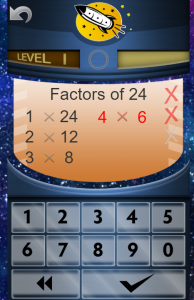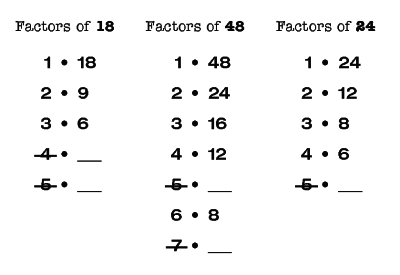## Foolproof method for finding factors

Knowing when you’ve found ALL the factors is the hard part.

Students have to learn how to find the factors of a number because several tasks in working with fractions require students to find the factors of numbers. Thinking of some of the factors of a number is not hard. What is hard is knowing when you have thought of ALL the factors. Here is a foolproof, systematic method I recommend: starting from 1 and working your way up the numbers. This is what student practice in the Worksheet Program Factors Learning Track.  Students also learn the pairs of factors in this sequence in the Online Game.Dr Don has a white board type video lesson that explains this in 6 minutes.

https://www.educreations.com/lesson/view/how-to-find-all-the-factors-of-a-number/46790401/

How to find all the factors of numbers
Always begin with 1 and the number itself-those are the first two factors. You write 1 x the number.  Then go on to 2. Write that under the 1. If the number you are finding factors for is an even number then 2 will be a factor. Think to yourself “2 times what equals the number we are factoring?” The answer will be the other factor.
However, if the number you are finding factors for is an odd number, then 2 will not be a factor and so you cross it out and go on to 3. Think to yourself “3 times what equals the number we are factoring?” There’s no easy rule for 3s like there is for 2s. But if you know the multiplication facts you will know if there is something. Then you go on to four—and so on.

The numbers on the left start at 1 and go up in value.  The numbers on the right go down in value.  You know you are done when you come to a number on the left that you already have on the right.  Let’s try an example.Let’s find the factors of 18.  (To the left you see a part of a page from the Rocket Math factoring program.)
We start with the first two factors, 1 and 18. We know that one times any number equals itself. We write those down.
Next we go to 2. 18 is an even number, so we know that 2 is a factor. We say to ourselves, “2 times what number equals 18?” The answer is 9. Two times 9 is 18, so 2 and 9 are factors of 18.
Next we go to 3. We say to ourselves, “3 times what number equals 18?” The answer is 6. Three times 6 is 18, so 3 and 6 are factors of 18.
Next we go to 4. We say to ourselves, “4 times what number equals 18?” There isn’t a number. We know that 4 times 4 is 16 and 4 times 5 is 20, so we have skipped over 18. We cross out the 4 because it is not a factor of 18.
Next we go to 5. We might say to ourselves, “5 times what number equals 18?” But we know that 5 is not a factor of 18 because 18 does not end in 5 or 0 and only numbers that end in 5 and 0 have 5 as a factor. So we cross out the five.
We would next go to 6, but we don’t have to. If we look up here on the right side we see that 6 is already identified as a factor. So we have identified all the factors there are for 18. Any more factors that are higher we have already found. So we are done.

Now let’s do another number.  Let’s find the factors of 48.

We start with the first two factors, 1 and 48.  We know that one times any number equals itself.

Next we go to 2.  48 is an even number, so we know that 2 is a factor.  We say to ourselves, “2 times what number equals 48?”  We might have to divide 2 into 48 to find the answer is 24.  But yes 2 and 24 are factors of 48.

Next we go to 3.  We say to ourselves, “3 times what number equals 48?”   The answer is 16.  We might have to divide 3 into 48 to find the answer is 16.  But yes 3 and 16 are factors of 48.

Next we go to 4.  We say to ourselves, “4 times what number equals 48?”  If we know our 12s facts we know that 4 times 12 is 48.  So 4 and 12 are factors of 48.

Next we go to 5.  We might say to ourselves, “5 times what number equals 48?”   But we know that 5 is not a factor of 48 because 48 does not end in 5 or 0 and only numbers that end in 5 and 0 have 5 as a factor. So we cross out the five.

Next we go to 6. We say to ourselves, “6 times what number equals 48?”  If we know our multiplication facts we know that 6 times 8 is 48.  So 6 and 8 are factors of 48.

Next we go to 7.   We say to ourselves, “7 times what number equals 48?”   There isn’t a number.  We know that 7 times 6 is 42 and 7 times 7 is 49, so we have skipped over 48.  We cross out the 7 because it is not a factor of 48.

We would next go to 8, but we don’t have to.  If we look up here on the right side we see that 8 is already identified as a factor.  So we have identified all the factors there are for 48.  Any more factors that are higher we have already found.  So we are done.